﻿ Neutrino Oscillations in Four-dimension Space

### Neutrino Oscillations in Four-dimension Space

Hossein RahbarOPEN ACCESSPEER-REVIEWED

## Neutrino Oscillations in Four-dimension Space

Hossein RahbarDepartment of Physics, Faculty of science, Mazandaran University, Babolsar, Iran

### Abstract

Friedmann -Robertson- Walker (FRW) background used to study the oscillations of neutrino in four-dimension space. The Cauchy-Lorentz and Gaussian distribution in the base of this background used to obtain the probability of the neutrino oscillations. Finally, we pay attention to the case of associated incoherence damping coefficient of oscillation between flavors.

• Rahbar, Hossein. "Neutrino Oscillations in Four-dimension Space." International Journal of Physics 2.2 (2014): 34-36.
• Rahbar, H. (2014). Neutrino Oscillations in Four-dimension Space. International Journal of Physics, 2(2), 34-36.
• Rahbar, Hossein. "Neutrino Oscillations in Four-dimension Space." International Journal of Physics 2, no. 2 (2014): 34-36.

 Import into BibTeX Import into EndNote Import into RefMan Import into RefWorks

### 1. Introduction

Quantum gravity contains incoherent microscopic models with stochastic metric. Decoherence appeared in these models have contributions from stochastic quantum Gravity, which is operating as an effective medium, as well as from conventional uncertainties in the energy of the neutrino (anti-neutrino) beam. All these contributions lead to damping factors modulating the oscillatory terms. In some theoretical models, the phenomenon of space-time may be incorporated . According to this picture, the singular microscopic fluctuations of the metric give the ground state of quantum gravity of the structure of a stochastic medium. The medium has the profound effect of CPT-violating  decoherence of quantum matter as it propagates. This may have experimentally observable consequences in principle . One of the basic effects of gravitational de-coherence is the presence of factors which damp oscillatory terms or coherence. Space-time foam models do not consist exclusively of microscopic black holes, although there are the most popular models, another types of foam, inspired by brain-world scenarios . We do not explain this subject here. The most important things here is that to discuss the neutrino oscillation.

The neutrino oscillation can be studied in the stochastic quantum gravity environment . Measurable quantities, such as transition amplitudes between neutrino flavors will be obtained by averaging over the stochastic variables. The influence of the quantum gravity environment has a de-coherence effect on the neutrino state which is equivalent to a damping of the oscillations between neutrino flavors . The determination of the particular statistical distribution for the random fluctuations of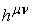requires complete knowledge of the quantum gravity theory. We extend this idea to more generic models of stochastically fluctuating metrics living in a full-fledged four-dimensional space time. We note here the allowed distributions corresponding characteristic function have a good results for physical observable (flavor oscillation probability). This criterion allows using this distribution in form of Cauchy-Lorentz and Gaussian. All above information about Cauchy-Lorentz and Gaussian distributions give us motivation to use Friedmann-Robertson Walker (FRW) metric. In this background we discuss the neutrino oscillations and compare the lamping aspect of waves in different distributation.

### 2. Flavor Oscillations in a Stochastic Metric Background

First we consider the FRW metric shown by the following expression :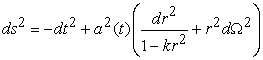(1)

By using the Einstein equation the corresponding Fridmann equations will be as [4, 5, 6],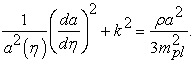And,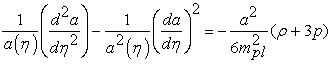(2)

Where,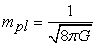is Plank mass and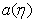is scale factor. This factor is a function of time and known as inflation (expansion) field of universe. p andare pressure and density respectively. k is curvature constant that describe the geometry of universe in three dimensional spaces.

In Friedmann model k = +1, 0, -1 , where k = 1 universe is hyper-spherical space, k=0 universe is flat and k = -1 universe is hyperbola. So, we have following relations for the density and pressure,(3)

Where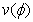and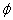are potential and field respectively. Friedmann equations in a flat space universe k = 0 are,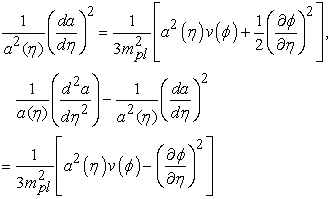(4)

For a interacting cosmic fluids in power-law Fridmann-Robertson-Walker cosmolog- ical model in flat space, a(t) can be obtained using Friedmann equations, continuity relations and the equation of states give us the following equation ,(5)

Whereis the constant of integration and can be obtained using proper initial conditions, and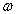is a constant related to shape of fluids. In case ofwe have matter-dominated universe, and pressure is very small compared with mass density. Therefore in matter-dominated case, one can obtain,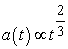(6)

Another important example is the case of radiation-dominated universe in which. In this case we have following expression,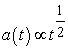(7)

To find the scalar particle in a state with flavor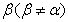, we use the probability relation [9, 15],(8)

The unitary matrix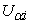correspond to the transformation from flavor eigenstates,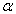to energy eigenstates i basis whereare frequency of oscillations between flavor states. To obtain oscillation probability, it is necessary to calculate average over(9)

Whereis multi variable probability density function.

Before solving this integral, it is necessary to obtain oscillation frequency, using Klein-Gordon equation in Fridmann-Robertson Walker metric.

The Klein-Gordon equation for a single scalar particle in a gravitational background is:(10)

Where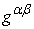, is the inverse metric tensor which can be define by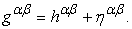And,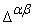is a gravitational covariant derivative, and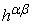is a flat fluctuation around background metric andis the Minkowski inverse metric which is considered as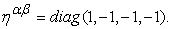By using the following metric,(11)

And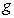considering flat Minkowski space-time background, the Klein-Gordon equation become,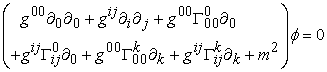(12)

Whereis the Christoffel symbol with following definition: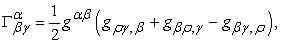(13)

And appeared in equation (13) can be define byTherefore nonzero elements of Christoffel symbol can be obtain by following relation:(14)

Where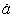is the derivative of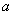respect to time. The solutions of Klein-Gordon equation will be as,(15)

By substitution of this equation in to (10), we obtain,(16)

By considering the first order of perturbation, one can obtainas,(17)

With an asymptotic conditionand first order approximation we obtain the following relation for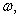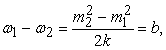(18)

In that case the average of exponential term will be as,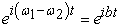(19)

Which play role phase in oscillation probability.

As a second order approximation in to (17), one obtains:(20)

Where A and B are:(21)

And the average of exponential term has a following expression(22)

With,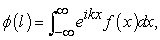(23)

Whereis the suitable probability density distribution function with x as a random variable.

To obtain oscillation probability, it is necessary to consider proper distribution function.

If we consider Gaussian distribution for the metric fluctuations, with the following density function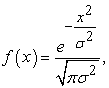(24)

Whereis the corresponding standard deviation of this distribution, we obtain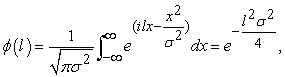(25)

Hence, the damping of oscillation probability of flavors become,(26)

WhereIn Cauchy-Lorentz distribution with following density distribution function,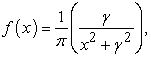(27)

And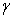as scale parameter we obtain,(28)

Therefore the oscillation probability become,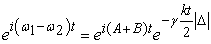(29)

Wherehas been defined previously.

### 3. Conclusions

In this paper, in order to investigate the oscillation probability we use two different distributaries. Also we employed Friedmann-Robinson-Walker metric in flat form. We have shown that the type of distribution strongly affected on damping of oscillation probability. In case of Cauchy-Lorentz distribution the damping of oscillation probability has an exponential damping linear in time and related to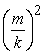. This exponential damping can be explained by de-coherent model of Lindblad [10, 11]. In the case of Gaussian distribution, the amplitude of flavor oscillation cause an exponential damping but it will be form of quadratic in time. The results obtained here are agreed with ref .

### References

  J. A. Wheeler and K. Ford, Geons, "Black Holes and Quantum foam" A life in physics (1998) and references therein.In article  R. M. Wald,Phys.Rev.D 21 (1980) 2742.In article CrossRef  N. E. Mavromatos, "lecture notes in physics", vol. 669, springer-verleg, Berlin (2005) p. 245.In article  Y. Tanaka, Chaos, Soliton and Fractals 23 (2005) 33-41.In article CrossRef  Y. Tanaka, Y. Mizuno and T. Kado, Chaos, Soliton and Fractals 24 (2005) 407-422.In article CrossRef  Y. Tanaka, Y. Mizuno T. Kado and H. A. Zhao, Chaos Soliton and Fractals 31 (2007) 1054-1075.In article CrossRef  P. C. Stavrinos, A. P. Kouretsis and M. Stathakopoulos, Gen Relativ Gravit 40 (2008)1403-1425.In article CrossRef  A.Studenikin and A.Ternov, Phys.Lett.B, 608,107(2005), hep-ph/0412408; E.Lobanov, Phys.Lett.B, 608(2005) 136.In article  V.B.Berestetskii, E.M.Lifschitz and P.Pitaevskii, "Quantum electrodynamics" (Moscow, Nauka, 1989), pp.108-112.In article  C.S.Lim and W.J.Marciano, Phys.Rev.D 37 (1988)1368.In article CrossRef  See pp.477-478 in Ref..In article  F. Benatti and R. Floreanini, Phys. Rev. D 64 (2001) 085015.In article CrossRef  N. E. Mavromatos and S. Sarkar, Phys. Rev. D 74 (2006) 036007.In article CrossRef  D. Oriti, arXiv:gr-qc/0311066v1 (2003).In article  M. Dvornikov, J. Maalampi, arxiv: 0809. 0963v1[hep-ph]5 sep 2008.In article  H.Maiwa and S.Naka, arXiv:hep-ph/0401143v3 (2004).In article  N. D. Birrell and P. c. W. Davies, Cambridge, UK: University press (1982) p 340.In article